# adding values to the last 10 bars

##### New member
Hi,
Need help coding the following scenario:

input length = 10;
I want to calculate Exp(-(Power(-i, 2) / 128)) for the last X bars
I would like to see in my last 10 bars the following values:

mydef = 8.173035134
mydef = 8.634157081
mydef = 8.996859656
mydef = 9.247021397
mydef = 9.374678698
mydef = 9.374678698
mydef = 9.247021397
mydef = 8.996859656
mydef = 8.634157081
mydef = 8.173035134

I am trying to do a loop to get the first value of 8.173035134

counter result
0 1
-1 0.992217938
-2 0.969233234
-3 0.932102492
-4 0.882496903
-5 0.822577562
-6 0.754839602
-7 0.681940751
-8 0.60653066
-9 0.531095991

and so for

Can you tell me what I am doing wrong?

This is my code:

declare lower;

input length = 10;
def lastBar = HighestAll(if !IsNaN(close) then BarNumber() else 0);

plot mydef = if lastBar - length < BarNumber() then
fold i = 0 to length - 1 do
(
(fold j = 0 to length with s = 0 do
s + Exp(-(Power(i - j, 2) / 128)))
)
else 0;

AddLabel(yes, "mydef: " + mydef, Color.CYAN);
AddLabel(yes, "mydef: " + mydef, Color.CYAN);
AddLabel(yes, "mydef: " + mydef, Color.CYAN);
AddLabel(yes, "mydef: " + mydef, Color.CYAN);
AddLabel(yes, "mydef: " + mydef, Color.CYAN);
AddLabel(yes, "mydef: " + mydef, Color.CYAN);
AddLabel(yes, "mydef: " + mydef, Color.CYAN);
AddLabel(yes, "mydef: " + mydef, Color.CYAN);
AddLabel(yes, "mydef: " + mydef, Color.CYAN);

All my values for mydef are 8.634157081

All loop values:

 loop 1 loop 2 result Total 0​ 0​ 1​ -1​ 0.992218​ -2​ 0.969233​ -3​ 0.932102​ -4​ 0.882497​ -5​ 0.822578​ -6​ 0.75484​ -7​ 0.681941​ -8​ 0.606531​ -9​ 0.531096​ 8.173035​ 1​ 1​ 0.992218​ 0​ 1​ -1​ 0.992218​ -2​ 0.969233​ -3​ 0.932102​ -4​ 0.882497​ -5​ 0.822578​ -6​ 0.75484​ -7​ 0.681941​ -8​ 0.606531​ 8.634157​ 2​ 2​ 0.969233​ 1​ 0.992218​ 0​ 1​ -1​ 0.992218​ -2​ 0.969233​ -3​ 0.932102​ -4​ 0.882497​ -5​ 0.822578​ -6​ 0.75484​ -7​ 0.681941​ 8.99686​ 3​ 3​ 0.932102​ 2​ 0.969233​ 1​ 0.992218​ 0​ 1​ -1​ 0.992218​ -2​ 0.969233​ -3​ 0.932102​ -4​ 0.882497​ -5​ 0.822578​ -6​ 0.75484​ 9.247021​ 4​ 4​ 0.882497​ 3​ 0.932102​ 2​ 0.969233​ 1​ 0.992218​ 0​ 1​ -1​ 0.992218​ -2​ 0.969233​ -3​ 0.932102​ -4​ 0.882497​ -5​ 0.822578​ 9.374679​ 5​ 5​ 0.822578​ 4​ 0.882497​ 3​ 0.932102​ 2​ 0.969233​ 1​ 0.992218​ 0​ 1​ -1​ 0.992218​ -2​ 0.969233​ -3​ 0.932102​ -4​ 0.882497​ 9.374679​ 6​ 6​ 0.75484​ 5​ 0.822578​ 4​ 0.882497​ 3​ 0.932102​ 2​ 0.969233​ 1​ 0.992218​ 0​ 1​ -1​ 0.992218​ -2​ 0.969233​ -3​ 0.932102​ 9.247021​ 6​ 7​ 0.681941​ 6​ 0.75484​ 5​ 0.822578​ 4​ 0.882497​ 3​ 0.932102​ 2​ 0.969233​ 1​ 0.992218​ 0​ 1​ -1​ 0.992218​ -2​ 0.969233​ 8.99686​ 8​ 8​ 0.606531​ 7​ 0.681941​ 6​ 0.75484​ 5​ 0.822578​ 4​ 0.882497​ 3​ 0.932102​ 2​ 0.969233​ 1​ 0.992218​ 0​ 1​ -1​ 0.992218​ 8.634157​ 9​ 9​ 0.531096​ 8​ 0.606531​ 7​ 0.681941​ 6​ 0.75484​ 5​ 0.822578​ 4​ 0.882497​ 3​ 0.932102​ 2​ 0.969233​ 1​ 0.992218​ 0​ 1​ 8.173035​

Last edited:
Hi,
Need help coding the following scenario:

input length = 10;
I want to calculate Exp(-(Power(-i, 2) / 128)) for the last X bars
I would like to see in my last 10 bars the following values:

mydef = 8.173035134
mydef = 8.634157081
mydef = 8.996859656
mydef = 9.247021397
mydef = 9.374678698
mydef = 9.374678698
mydef = 9.247021397
mydef = 8.996859656
mydef = 8.634157081
mydef = 8.173035134

there is nothing in your fold loops to alter the output.
on each bar, the loop will run through 10 iterations of the do formula and add up the same values.

why do you want to see those 10 numbers?

can you explain in math, and not coding, how you calculated those 10 numbers.
i can't create them.
can you make a long addition formula that creates the numbers?

what is the last length value? is it some number that is supposed to be changing ?
you have a constant variable , called length. that is always = 10.

there is no reason to show a number table of a bunch of bad numbers. if your fold doesn't work, it doesn't work.

Thank you for you replay and the time you took to help me.

I want to accomplish the following:
for the last ten bars I want to have the following numbers
mydef = 8.173035134
mydef = 8.634157081
mydef = 8.996859656
mydef = 9.247021397
mydef = 9.374678698
mydef = 9.374678698
mydef = 9.247021397
mydef = 8.996859656
mydef = 8.634157081
mydef = 8.173035134

how to get the number?

This is the loops that I used to work outside Thinkscript:
for k = 0 to length - 1
for m = 0 to length - 1
xxx = math.exp(-(math.pow(k-m,2)/128))

math.exp(-(math.pow(0,2)/128)) = 1
math.exp(-(math.pow(-1,2)/128)) = 0.992218
math.exp(-(math.pow(-2,2)/128)) = 0.969233
math.exp(-(math.pow(-3,2)/128)) = 0.932102
math.exp(-(math.pow(-4,2)/128)) = 0.882497
math.exp(-(math.pow(-5,2)/128)) = 0.822578
math.exp(-(math.pow(-6,2)/128)) = 0.75484
math.exp(-(math.pow(-7,2)/128)) = 0.681941
math.exp(-(math.pow(-8,2)/128)) = 0.606531
math.exp(-(math.pow(-9,2)/128)) = 0.531096

After adding the first set the total is 8.173035134
This should be the value for my last bar

The previous bar will run from 1 to -8
math.exp(-(math.pow(1,2)/128)) = 0.992218
math.exp(-(math.pow(0,2)/128)) = 1
math.exp(-(math.pow(-1,2)/128)) = 0.992218
math.exp(-(math.pow(-2,2)/128)) = 0.969233
math.exp(-(math.pow(-3,2)/128)) = 0.932102
math.exp(-(math.pow(-4,2)/128)) = 0.882497
math.exp(-(math.pow(-5,2)/128)) = 0.822578
math.exp(-(math.pow(-6,2)/128)) = 0.75484
math.exp(-(math.pow(-7,2)/128)) = 0.681941
math.exp(-(math.pow(-8,2)/128)) = 0.606531

After adding the second set the total is 8.634157

The previous bar will run from 2 to -7
math.exp(-(math.pow(2,2)/128)) = 0.969233
math.exp(-(math.pow(1,2)/128)) = 0.992218
math.exp(-(math.pow(0,2)/128)) = 1
math.exp(-(math.pow(-1,2)/128)) = 0.992218
math.exp(-(math.pow(-2,2)/128)) = 0.969233
math.exp(-(math.pow(-3,2)/128)) = 0.932102
math.exp(-(math.pow(-4,2)/128)) = 0.882497
math.exp(-(math.pow(-5,2)/128)) = 0.822578
math.exp(-(math.pow(-6,2)/128)) = 0.75484
math.exp(-(math.pow(-7,2)/128)) = 0.681941

After adding the 3 set the total is 8.99686
and so for

Thank you for you replay and the time you took to help me.

This is the loops that I used to work outside Thinkscript:
for k = 0 to length - 1
for m = 0 to length - 1
xxx = math.exp(-(math.pow(k-m,2)/128))

EDIT 2
reading post 15 a few times,
1 fold loop will work , by adding in the difference of current barnumber to the last barnumber, over the last 10 bars

----------------------------------------
EDIT , updated
i was trying to post something, on my phone, as i was going out the door,....
sorry, i should have just waited .
here is the link to a study that uses a nested loop.

i will experiment with your study in a bit
-----------------
those 2 for loops make sense . That's what I needed.
I will work on it later.

i had posted this, but it doesn't apply.
i'll leave it because it has nested fold loops

Last edited:

•halcyonguy
in the example I use the length of 10, but can apply for all the candles

•halcyonguy
Thank you for you replay and the time you took to help me.

I want to accomplish the following:
for the last ten bars I want to have the following numbers
mydef = 8.173035134
mydef = 8.634157081
mydef = 8.996859656
mydef = 9.247021397
mydef = 9.374678698
mydef = 9.374678698
mydef = 9.247021397
mydef = 8.996859656
mydef = 8.634157081
mydef = 8.173035134

how to get the number?

This is the loops that I used to work outside Thinkscript:
for k = 0 to length - 1
for m = 0 to length - 1
xxx = math.exp(-(math.pow(k-m,2)/128))

math.exp(-(math.pow(0,2)/128)) = 1
math.exp(-(math.pow(-1,2)/128)) = 0.992218
math.exp(-(math.pow(-2,2)/128)) = 0.969233
math.exp(-(math.pow(-3,2)/128)) = 0.932102
math.exp(-(math.pow(-4,2)/128)) = 0.882497
math.exp(-(math.pow(-5,2)/128)) = 0.822578
math.exp(-(math.pow(-6,2)/128)) = 0.75484
math.exp(-(math.pow(-7,2)/128)) = 0.681941
math.exp(-(math.pow(-8,2)/128)) = 0.606531
math.exp(-(math.pow(-9,2)/128)) = 0.531096

After adding the first set the total is 8.173035134
This should be the value for my last bar

The previous bar will run from 1 to -8
math.exp(-(math.pow(1,2)/128)) = 0.992218
math.exp(-(math.pow(0,2)/128)) = 1
math.exp(-(math.pow(-1,2)/128)) = 0.992218
math.exp(-(math.pow(-2,2)/128)) = 0.969233
math.exp(-(math.pow(-3,2)/128)) = 0.932102
math.exp(-(math.pow(-4,2)/128)) = 0.882497
math.exp(-(math.pow(-5,2)/128)) = 0.822578
math.exp(-(math.pow(-6,2)/128)) = 0.75484
math.exp(-(math.pow(-7,2)/128)) = 0.681941
math.exp(-(math.pow(-8,2)/128)) = 0.606531

After adding the second set the total is 8.634157

The previous bar will run from 2 to -7
math.exp(-(math.pow(2,2)/128)) = 0.969233
math.exp(-(math.pow(1,2)/128)) = 0.992218
math.exp(-(math.pow(0,2)/128)) = 1
math.exp(-(math.pow(-1,2)/128)) = 0.992218
math.exp(-(math.pow(-2,2)/128)) = 0.969233
math.exp(-(math.pow(-3,2)/128)) = 0.932102
math.exp(-(math.pow(-4,2)/128)) = 0.882497
math.exp(-(math.pow(-5,2)/128)) = 0.822578
math.exp(-(math.pow(-6,2)/128)) = 0.75484
math.exp(-(math.pow(-7,2)/128)) = 0.681941

After adding the 3 set the total is 8.99686
and so for

Ruby:
``````# power_loop_01
# post13
# ----------------------------
# use 1 loop and offset to last bar

def na = Double.NaN;
def bn = barnumber();
def lastbar = !isnan(close) and isnan(close[-1]);
input length = 10;
def lastbn = if bn == 1 then 0
else if ( !isnan(close[-(length-1) ]) and isnan(close[-length]) ) then (bn + length)
else lastbn;

#  true during the last 'length' bars on chart
def lastxbars = if (bn >= (lastbn - length)) and bn <= lastbn then 1 else 0;

#  count down numbers
def start = if lastxbars then ((lastbn - bn)- length ) else na;
def end = start + length - 1;

# run a loop 'length' times and add up the numbers , for each bar near the last bar
def mydef;
if lastxbars then {
mydef = fold i = start to end+1
with p
do p + Exp(-(Power(i, 2) / 128));
} else {
mydef = 0;
}

def labelon = lastbar;
AddLabel(labelon, "mydef: " + mydef, Color.CYAN);
AddLabel(labelon, "mydef: " + mydef, Color.CYAN);
AddLabel(labelon, "mydef: " + mydef, Color.CYAN);
AddLabel(labelon, "mydef: " + mydef, Color.CYAN);
AddLabel(labelon, "mydef: " + mydef, Color.CYAN);
AddLabel(labelon, "mydef: " + mydef, Color.CYAN);
AddLabel(labelon, "mydef: " + mydef, Color.CYAN);
AddLabel(labelon, "mydef: " + mydef, Color.CYAN);
AddLabel(labelon, "mydef: " + mydef, Color.CYAN);
AddLabel(labelon, "mydef: " + mydef, Color.CYAN);

# --------------------------
# test data

input show_test_bubbles = yes;
bn + " bn\n" +
lastbn + " last\n" +
start + " start\n" +
end + " end\n" +
mydef
, color.yellow, no);
#
#``````

fold loops don't process the final to value.
if you want to include that number ( end ) then add 1 to it
mydef = fold i = start to end+1

length = 10
10 labels of numbers
10 bubbles showing some values, on last 10 bars
the \n in the bubble text, places text after it, to be on a new line.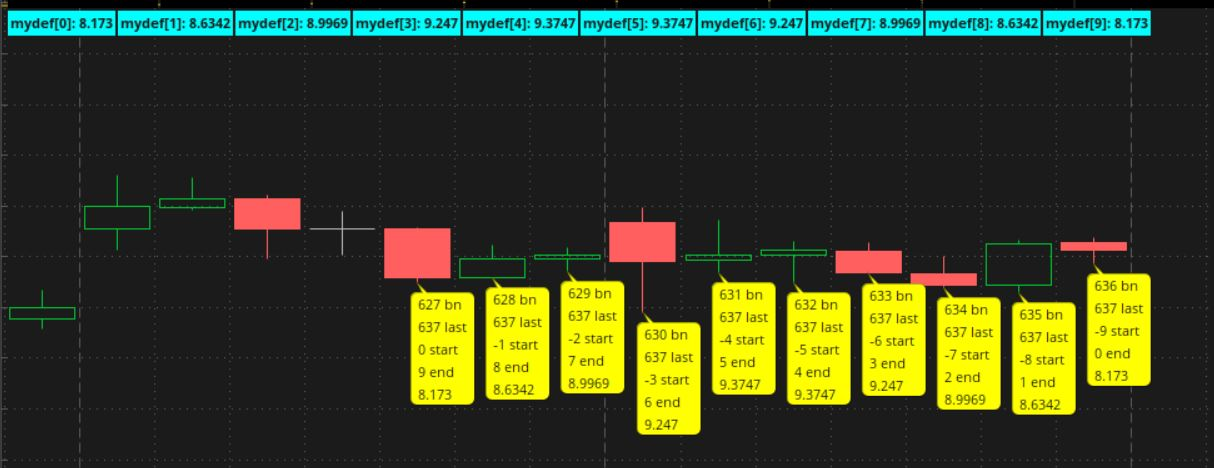Last edited:
Incredible jobI will continue with the conversion

•halcyonguy
I like the way you test the data

@halcyonguy

I have a challenge and I hope the last one in this project is to add the close price to the equation.
I need to keep the previous loop as well. basically I need 2 loops.
One for Exp and one for Exp * Price
I was thinking the loop will runs from 0 to length.
You did an excellent job adjusting the numbers in the loop to create a sigle (not a nested) one.

def myPricedef;
if lastxbars then {
myPricedef = fold j = start to end + 1
with p2
do p2 + GetValue(close,j)*Exp(-(Power(j, 2) / 128));
} else {
myPricedef = 0;
}

The problem I am facing is that I need the GetValue(close,from 0 to length-1) all the time while Exp(-(Power(j, 2) / 128)) will range from the values you set

*************************************************************
This the code I am trying to convert
*************************************************************
for i = 0 to length-1
sum = 0.
sumw = 0.

for j = 0 to length-1
w = math.exp(-(math.pow(i-j,2)/128))
sum += src[j]*w
sumw += w
*************************************************************

Trying to figure it out

EDIT
GetValue and Exp indexes must be in sync to add more troubles to the solution
-----------------------------------------------------------------------------------------

Last edited:
Working in this solution to see if helps

def sum;
if lastxbars then {
sum = fold j = start to end + 1
with p2
do p2 + GetValue(close,-(j-end))*Exp(-(Power(j, 2) / 128));
} else {
sum = 0;
}

@halcyonguy

I have a challenge and I hope the last one in this project is to add the close price to the equation.
I need to keep the previous loop as well. basically I need 2 loops.
One for Exp and one for Exp * Price
I was thinking the loop will runs from 0 to length.
You did an excellent job adjusting the numbers in the loop to create a sigle (not a nested) one.

def myPricedef;
if lastxbars then {
myPricedef = fold j = start to end + 1
with p2
do p2 + GetValue(close,j)*Exp(-(Power(j, 2) / 128));
} else {
myPricedef = 0;
}

The problem I am facing is that I need the GetValue(close,from 0 to length-1) all the time while Exp(-(Power(j, 2) / 128)) will range from the values you set

*************************************************************
This the code I am trying to convert
*************************************************************
for i = 0 to length-1
sum = 0.
sumw = 0.

for j = 0 to length-1
w = math.exp(-(math.pow(i-j,2)/128))
sum += src[j]*w
sumw += w
*************************************************************

Trying to figure it out

EDIT
GetValue and Exp indexes must be in sync to add more troubles to the solution
-----------------------------------------------------------------------------------------

i didn't see post 24 till i was about to post this

see if this is close to what you expected.
maybe it will be something for you to experiment with.

i am not sure what this means,
. the GetValue(close,from 0 to length-1) all the time

i added ver2 code to the end of ver1
can't do close[-number] on the last bar, future bars don't exist yet.
i used absolute values of the loop counter numbers

do p2 + ( getvalue(close, absvalue(i2)) * Exp(-(Power(i2, 2) / 128)));

Ruby:
``````# power_loop_02

#--------------------------------------------
# ----------------------------
# post13

# ----------------------------
# use 1 loop and offset to last bar

def na = Double.NaN;
def bn = barnumber();
def lastbar = !isnan(close) and isnan(close[-1]);
input length = 10;
def lastbn = if bn == 1 then 0
else if ( !isnan(close[-(length-1) ]) and isnan(close[-length]) ) then (bn + length)
else lastbn;

#  true during the last 'length' bars on chart
def lastxbars = if (bn >= (lastbn - length)) and bn <= lastbn then 1 else 0;

#  count down numbers
def start = if lastxbars then ((lastbn - bn)- length ) else na;
def end = start + length - 1;

# run a loop 'length' times and add up the numbers , for each bar near the last bar
def mydef;
if lastxbars then {
mydef = fold i = start to end+1
with p
do p + Exp(-(Power(i, 2) / 128));
} else {
mydef = 0;
}

def labelon = lastbar;
AddLabel(labelon, "mydef: " + mydef, Color.CYAN);
AddLabel(labelon, "mydef: " + mydef, Color.CYAN);
AddLabel(labelon, "mydef: " + mydef, Color.CYAN);
AddLabel(labelon, "mydef: " + mydef, Color.CYAN);
AddLabel(labelon, "mydef: " + mydef, Color.CYAN);
AddLabel(labelon, "mydef: " + mydef, Color.CYAN);
AddLabel(labelon, "mydef: " + mydef, Color.CYAN);
AddLabel(labelon, "mydef: " + mydef, Color.CYAN);
AddLabel(labelon, "mydef: " + mydef, Color.CYAN);
AddLabel(labelon, "mydef: " + mydef, Color.CYAN);

# --------------------------
# test data

input show_test_bubbles = yes;
bn + " bn\n" +
lastbn + " last\n" +
start + " start\n" +
end + " end\n" +
mydef
, color.yellow, no);

# ------------------------------
# ref

# 1st sum value = 0 to -9

# 2nd sum value = 1 to -8
#The previous bar will run from 1 to -8
#math.exp(-(math.pow(1,2)/128)) = 0.992218
#math.exp(-(math.pow(0,2)/128)) = 1
#math.exp(-(math.pow(-1,2)/128)) = 0.992218
#math.exp(-(math.pow(-2,2)/128)) = 0.969233
#math.exp(-(math.pow(-3,2)/128)) = 0.932102
#math.exp(-(math.pow(-4,2)/128)) = 0.882497
#math.exp(-(math.pow(-5,2)/128)) = 0.822578
#math.exp(-(math.pow(-6,2)/128)) = 0.75484
#math.exp(-(math.pow(-7,2)/128)) = 0.681941
#math.exp(-(math.pow(-8,2)/128)) = 0.606531

# 3rd sum value = 2 to -7
# 4th sum value = 3 to -6
#

# ------------------------------------------------------------
# ------------------------------------------------------------

# ver 2
# post23

# The problem I am facing is that I need
#  the GetValue(close,from 0 to length-1) all the time while Exp(-(Power(j, 2) / 128))
#   will range from the values you set
# *************************************************************
# This the code I am trying to convert
# *************************************************************
# for i = 0 to length-1
# sum = 0.
# sumw = 0.

# for j = 0 to length-1
# w = math.exp(-(math.pow(i-j,2)/128))
# sum += src[j]*w
# sumw += w
# *************************************************************

# add a 2nd loop to do this
#     # sum += src[j]*w

# run a loop 'length' times and add up the numbers , for each bar near the last bar
def mydef2;
if lastxbars then {
mydef2 = fold i2 = start to end+1
with p2
do p2 + ( getvalue(close, absvalue(i2)) * Exp(-(Power(i2, 2) / 128)));
} else {
mydef2 = 0;
}

# muliply prev data by close, using an offset of  absolute value of the loop counter
input show_test2_bubbles = yes;
round( mydef2,2)
, color.cyan, no);
#
#``````

new data , in light blue bubbles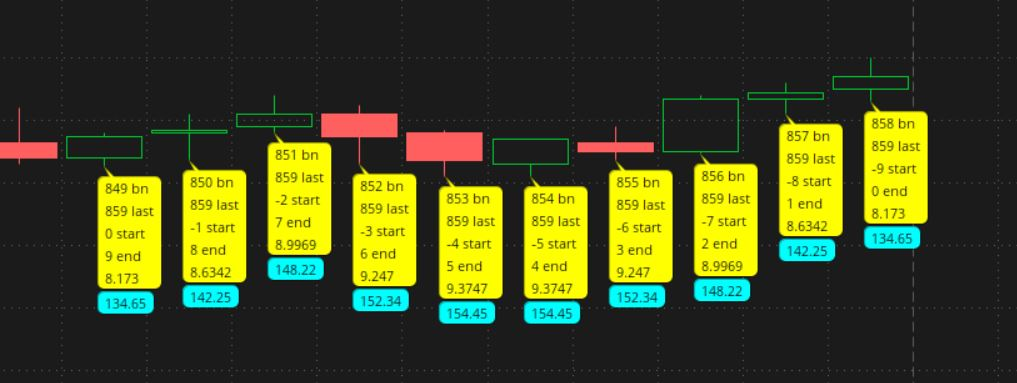did not see your code until now,
I came to the same code, after run some test,

Thank you million times

Can I insert an image here if I do a print screen?

So far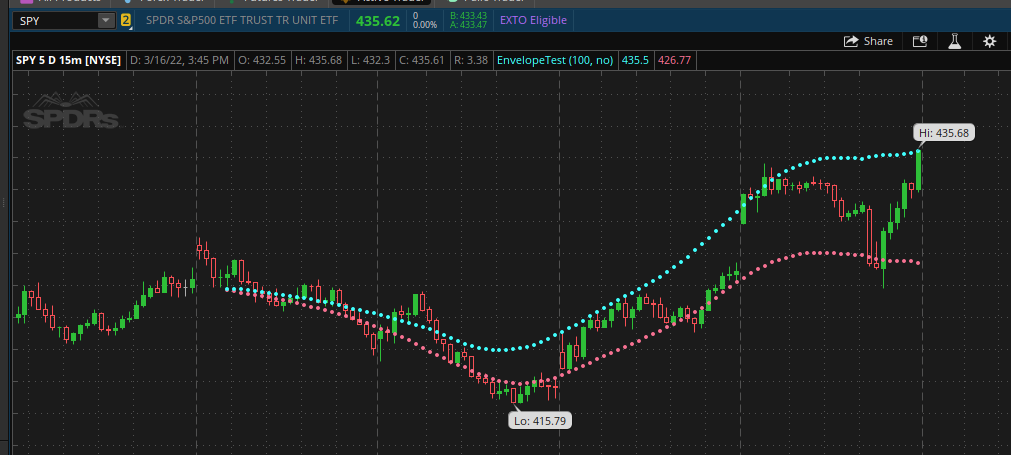versus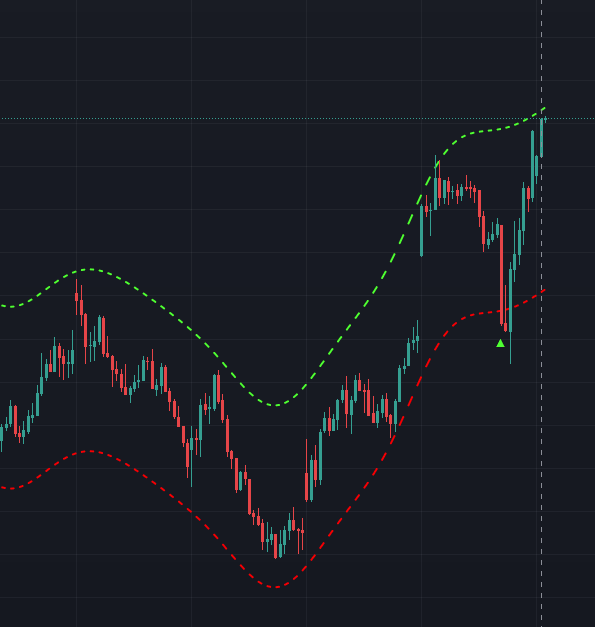•halcyonguy
can I ask you, what is the indicator you use the most and in what time frame?

this is the latest code I have

def na = Double.NaN;
def bn = barnumber();
def lastbar = !isnan(close) and isnan(close[-1]);
input length = 10;
def lastbn = if bn == 1 then 0
else if ( !isnan(close[-(length-1) ]) and isnan(close[-length]) ) then (bn + length)
else lastbn;

# true during the last 'length' bars on chart
def lastxbars = if (bn >= (lastbn - length)) and bn <= lastbn then 1 else 0;

# count down numbers
def start = if lastxbars then ((lastbn - bn)- length ) else na;
def end = start + length - 1;

# run a loop 'length' times and add up the numbers , for each bar near the last bar
def sumExp;
if lastxbars then {
sumExp = fold i = start to end + 1
with p1
do p1 + Exp(-(Power(i, 2) / 128));
} else {
sumExp = 0;
}

def sumExpWithPrice;
if lastxbars then {
sumExpWithPrice = fold j = start to end +1
with p2
do p2 + GetValue(close,-j)*Exp(-(Power(j, 2) / 128));
} else {
sumExpWithPrice = 0;
}

def y2 = if lastxbars then sumExpWithPrice/sumExp
else 0;

def sumAbs = if lastxbars then absvalue(close - y2)
else 0;

def mae = if lastxbars then TotalSum(sumAbs/length*3)
else 0;

plot upperPlot = if lastxbars then y2 + mae else na;
upperPlot.SetPaintingStrategy(PaintingStrategy.POINTS);

plot lowerPlot = if lastxbars then y2 - mae else na;
lowerPlot.SetPaintingStrategy(PaintingStrategy.POINTS);

#--------------------------
# test data

input show_test_bubbles = yes;
bn + " bn\n" +
lastbn + " last\n" +
start + " start\n" +
end + " end\n" +
y2 + " y2\n" +
sumAbs + " sumAbs\n" +
close + " close\n" +
mae + " mae"
, color.yellow, no);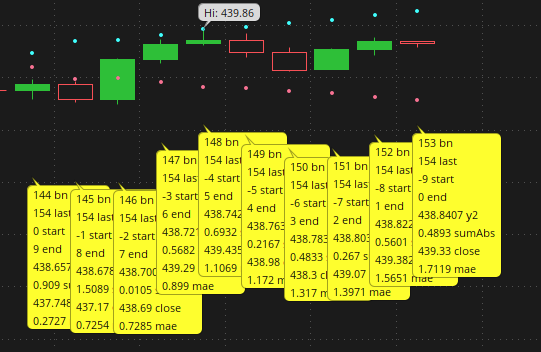Original values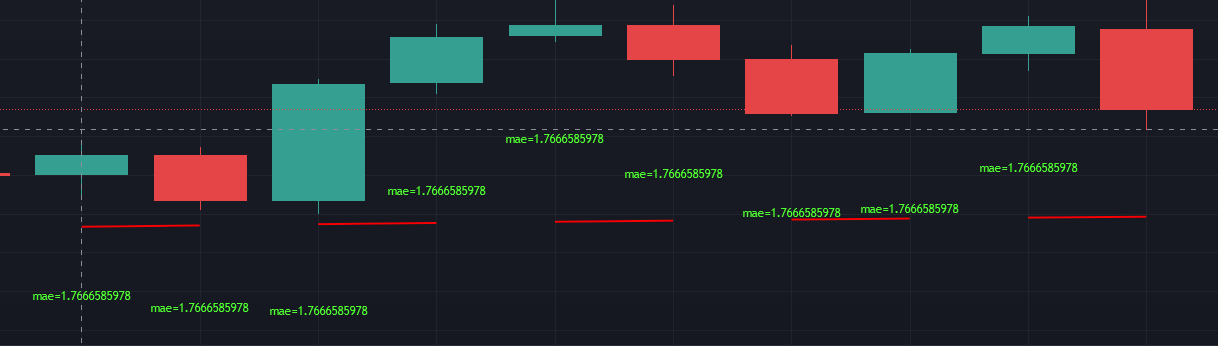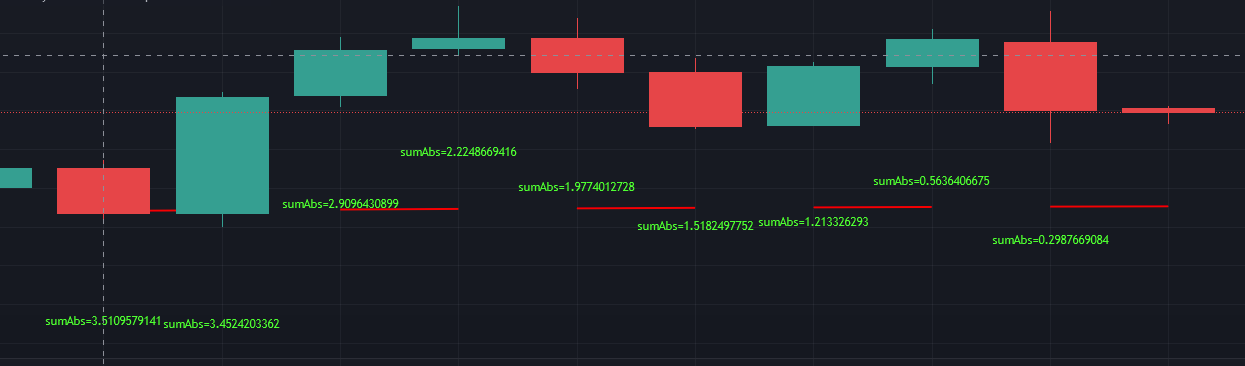The numbers does not need to match, but they more or less the same.
mae is unique for all the bars and the sumAbs is an accumulate number,

can't get the numbers to follow the the same logic
never imaging that will be so hard.

I used highestAll(mae) to get the max value

### Not the exact question you're looking for?

87k+ Posts
223 Online## The Market Trading Game Changer

Join 2,500+ subscribers inside the useThinkScript VIP Membership Club
• Exclusive indicators
• Proven strategies & setups
• Private Discord community
• Exclusive members-only content
• 1 full year of unlimited support

What is useThinkScript?

useThinkScript is the #1 community of stock market investors using indicators and other tools to power their trading strategies. Traders of all skill levels use our forums to learn about scripting and indicators, help each other, and discover new ways to gain an edge in the markets.

How do I get started?

We get it. Our forum can be intimidating, if not overwhelming. With thousands of topics, tens of thousands of posts, our community has created an incredibly deep knowledge base for stock traders. No one can ever exhaust every resource provided on our site.

If you are new, or just looking for guidance, here are some helpful links to get you started.

What are the benefits of VIP Membership?